## Monday, May 29, 2006

### Non-Relativistic QCD

This is another installment in our series about fermions on the lattice. In the previous posts in this series we had looked at various lattice discretisations of the continuum Dirac action, and how they dealt with the problem of doublers posed by the Nielsen-Ninomiya theorem. As it turned out, one of the main difficulties in this was maintaining chiral symmetry, which is important in the limit of vanishing quark mass. But what about the opposite limit -- the limit of infinite quark mass?

As it turns out, that limit is also difficult to handle, but for entirely different reasons: The correlation functions, from which the properties of bound states are extracted, show an exponential decay of the form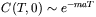, where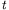is the number of timesteps, and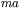is the product of the state's mass and the lattice spacing. Now for a heavy quark, e.g. a bottom, and the lattice spacings that are feasible with the biggest and fastest computers in existence today,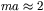, which means that the correlation functions for an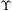will decay like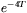, which is way too fast to extract a meaningful signal. (Making the lattice spacing smaller is so hard because in order to fill the same physical volume you need to increase the number of lattice points accordingly, which requires a large increase in computing power.)

Fortunately, in the case of heavy quark systems the kinetic energies of the heavy quarks are small compared to their rest masses, as evidenced by the relatively small splittings between the ground and excited states of heavy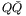mesons. This means that the heavy quarks are moving at non-relativistic velocities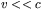and can hence be well described by a Schrödinger equation instead of the full Dirac equation after integrating out the modes with energies of the order of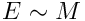. The corresponding effective field theory is known as Non-Relativistic QCD (NRQCD) and can be schematically written using the Lagrangian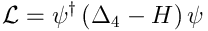where $\psi$ is a non-relativistic two-component Pauli spinor and the Hamiltonian is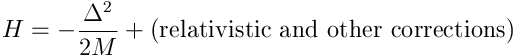In actual practice, this is not a useful way to write things, since it is numerically unstable for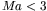; instead one uses an action that looks like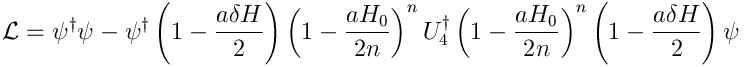where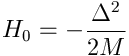whereas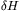incorporates the relativistic and other corrections, and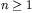is a numerical stability parameter that makes the system stable for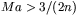.

This complicated form makes NRQCD rather formidable to work with, but it can be and has been successfully used in the description of thesystem and in other contexts. In fact, some of the most precise predictions from lattice QCD rely on NRQCD for the description of heavy quarks.

It should be noted that the covariant derivatives in NRQCD are nearest-neighbours differences -- the reasons for having to take symmetric derivatives don't apply in the non-relativistic case; hence there are no doublers in NRQCD.